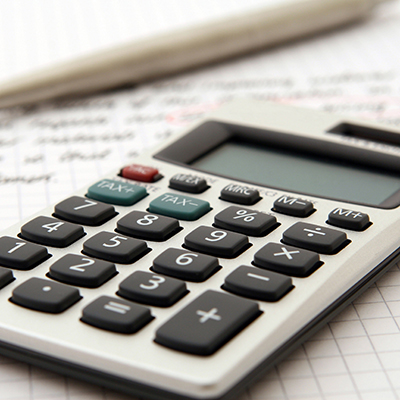Techniques and applications of integration; sequences, series, convergence tests, power series; parametric equations; polar coordinates.

#### Course Details

Note
Students will need access to WebAssign along with their textbook. Access can be purchased as a bundle with their textbook or individually.
Prerequisites
Math 112
Course Outline
Lesson 1: Area Between Curves
Lesson 2: Volumes
Lesson 3: Volumes by Cylindrical Shells
Lesson 4: Work
Lesson 5: Average Value of a Function
Lesson 6: Integration by Parts
Lesson 7: Trigonometric Integrals
Lesson 8: Trigonometric Substitution
Lesson 9: Integration of Rational Functions by Partial Fractions
Lesson 10: Strategy for Integration
Lesson 11: Approximate Integration
Lesson 12: Improper Integrals
Lesson 13: Arc Length
Lesson 14: Area of a Surface of Revolution
Lesson 15: Applications to Physics and Engineering
Lesson 16: Probability
Lesson 17: Curves Defined by Parametric Equations
Lesson 18: Calculus with Parametric Curves
Lesson 19: Polar Coordinates
Lesson 20: Calculus in Polar Coordinates
Lesson 21: Sequences
Lesson 22: Series
Lesson 23: The Integral Test and Estimates of Sums
Lesson 24: Comparison Tests
Lesson 25: Alternating Series and Absolute Convergence
Lesson 26: The Ratio and Root Tests
Lesson 27: Strategy for Testing Series
Lesson 28: Power Series
Lesson 29: Representation of Functions as Power Series
Lesson 30: Taylor and Maclaurin Series
Lesson 31: Applications of Taylor Polynomials
Syllabus
Textbooks
Amazon* Library
Single Variable Calculus, Early Transcendentals, Volume 2, 9th Edition, WITH WEBASSIGN (Required)

ISBN: 9780357631478
2021 Cengage
Used from \$136.93
New from \$134.58
Borrow
* As an Amazon Associate BYU Continuing Education earns from qualifying purchases.